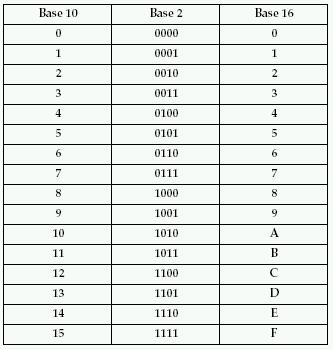#### The Hexadecimal Code

Think of the hexadecimal code as an extended binary code

Binary code is made up of sets of one bit (either 0 or 1) – LED ON or LED OFF

Hexadecimal code is made up of sets of four bits.

In general, computers use Assembly Language to carry out instructions.

Assembly language is virtually written entirely using hexadecimal code.

We can convert each set of four binary bits into a single hexadecimal bit.

This allows for more information to be stored and retrieved more efficiently.

Changing from binary [BASE 2] to hexadecimal [BASE 16] is called a four-bit conversion.

##### Comparing the number systems

Decimal

• ten possible digits (0 to 9)

Binary

• two  possible digits (0 and 1)

• sixteen possible digits (0 to 9 + A to F)
•

Converting BASE 2 to BASE 16

1. Divide binary numbers in groups of 4
2. Assign the hexadecimal value to each group
3. If a group does not have 4 digits add zeros in front of itExample 1: convert 1000111 into hexadecimal

1. First group = 0111
2. Second group = 0100
(noticed we added a 0 in front of the one)

Group 1

• 0111 = 7   (from the chart)

Group 2

• 0100 = 4

Therefore 1000111  = 47

Example 2: convert 101110101101 into hexadecimal

1011 1010 1101

1. First group = 1101
2. Second group = 1010
3. Third group = 1011

Group 1

• 0111 = “D”  (from the chart)

Group 2

• 1010 = “A”

Group 3

• 1010 = “B”

Therefore: